Related Articles
Multiple Density Plots with Pandas in Python
• Last Updated : 03 Jan, 2021

Multiple density plots are a great way of comparing the distribution of multiple groups in your data.  We can make multiple density plots using pandas plot.density() function. However, we need to convert data in a wide format if we are using the density function. Wide data represents different groups in different columns. We convert data in a wide format using Pandas pivot() function.

Let’s create the simple data-frame and then reshape it into a wide-format:

Example 1:

Here we are using this data set.

Step 1: Creating dataframe from data set.

## Python3

 `import` `pandas as pd`` ` `# creating a dataframe``df ``=` `pd.read_csv(r``"gapminder1.csv"``)``df.head()`

Output: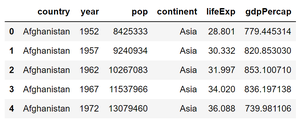dataset

Step 2: Let’s group data according to countries in different columns so that we can apply the density() function to plot multiple density plots.

## Python3

 `# converting data into wide-format``data_wide ``=` `df.pivot(columns``=``'continent'``,``                     ``values``=``'lifeExp'``)``data_wide.head()`

Output: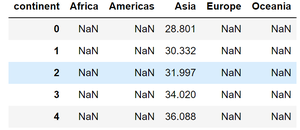Step 3: Now let’s plot multiple density plot using plot.density()

## Python3

 `import` `matplotlib.pyplot as plt`` ` `# calling density() to make``# multiple density plot ``data_wide.plot.density(figsize ``=` `(``7``, ``7``),``                       ``linewidth ``=` `4``)`` ` `plt.xlabel(``"life_Exp"``)`

Output :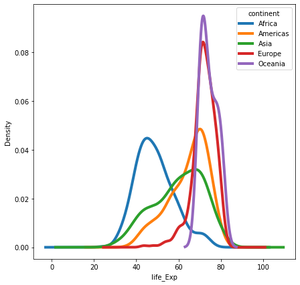Multiple density plots

Example 2: We can also call plot.kde() function on dataframe to make multiple density plots with Pandas.

Here we are using the tips dataset for this example, You can find it here.

Step 1: Creating dataframe from data set.

## Python3

 `import` `pandas as pd`` ` `# creating a dataframe``df ``=` `pd.read_csv(r``"tips.csv"``)``df.head()`

Output: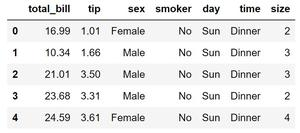tips_df

Step 2: Now apply pivot() function to have dataframe in the wide-format then apply kde() to have multiple density plot.

## Python3

 `# Converting to wide dataframe``data_wide ``=` `df.pivot(columns ``=` `'day'``,``                     ``values ``=` `'total_bill'``)`` ` `# plotting multiple density plot``data_wide.plot.kde(figsize ``=` `(``8``, ``6``),``                   ``linewidth ``=` `4``)`

Output: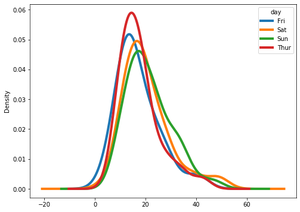tips multiple D.P

Attention geek! Strengthen your foundations with the Python Programming Foundation Course and learn the basics.

To begin with, your interview preparations Enhance your Data Structures concepts with the Python DS Course.

My Personal Notes arrow_drop_up